# 高雄 城市商旅【真愛館】 City Suites Chenai

1,650

• 駁二藝術特區
• 真愛碼頭
• 捷運鹽埕埔站O2

## 選擇專案房型及間數

#### 更改 高雄 城市商旅【真愛館】 的空房情形及價格

•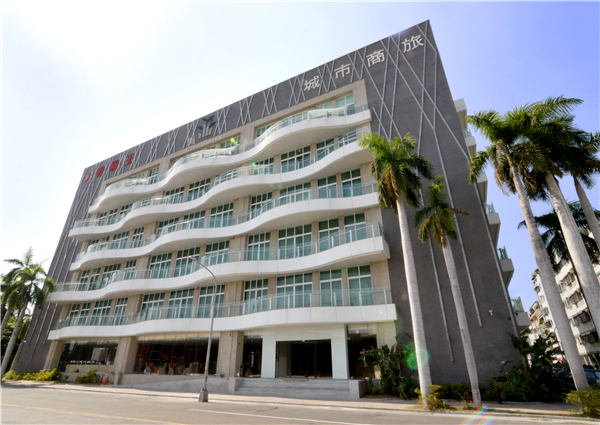酒店外觀
•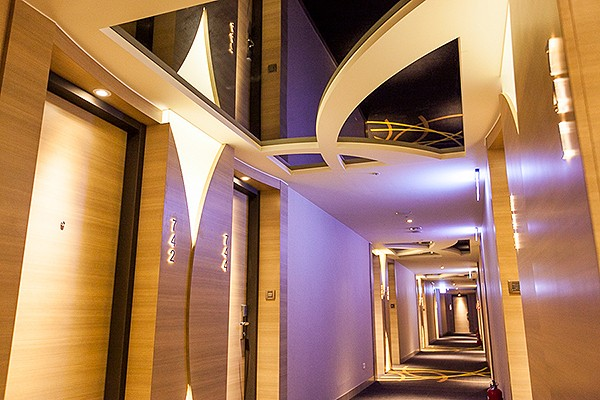環境
•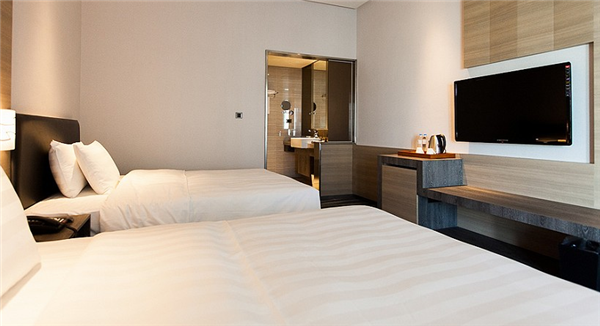城市客房
•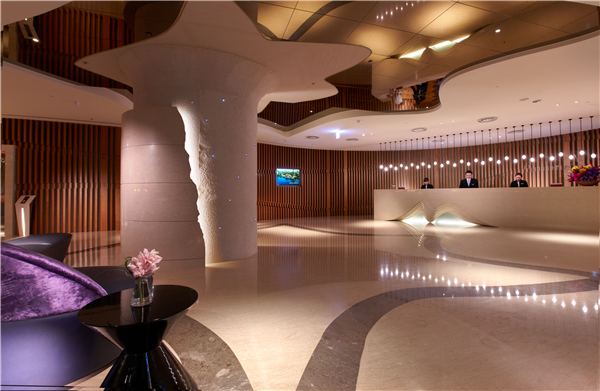大廳
•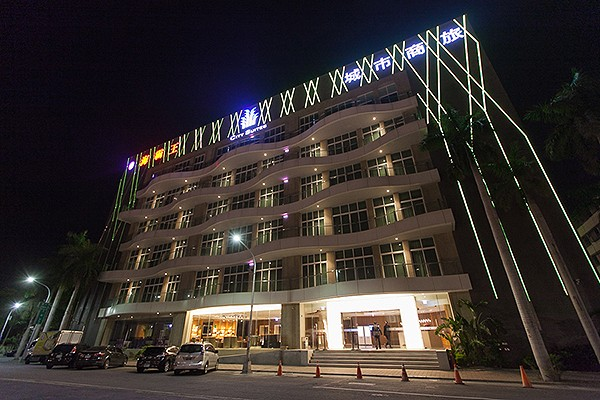環境
•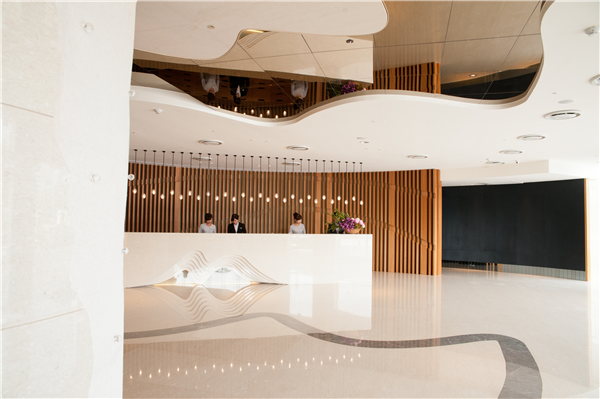大廳
•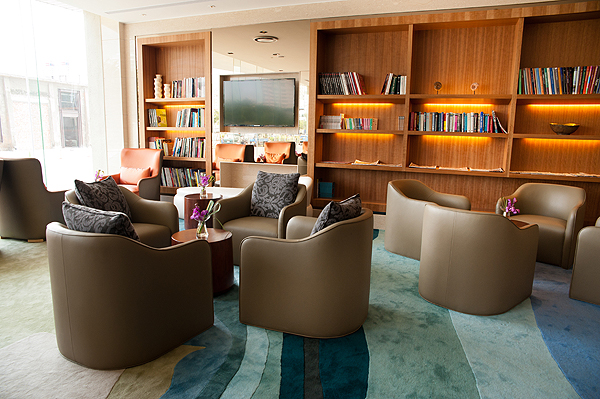環境
•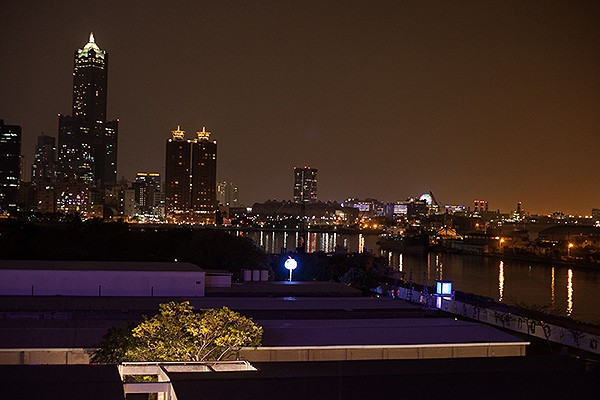環境
•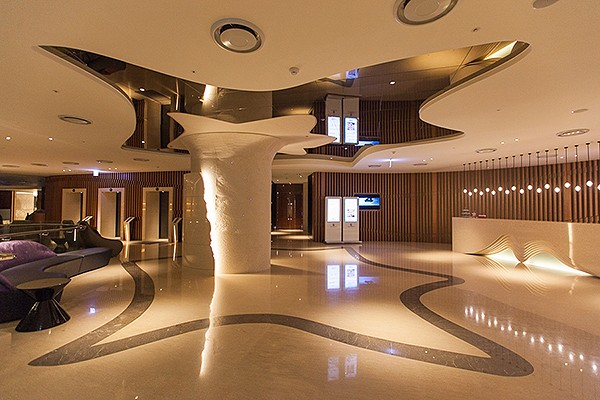環境
•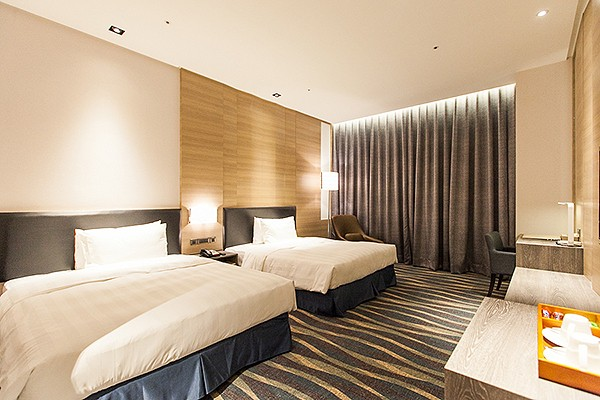環境
•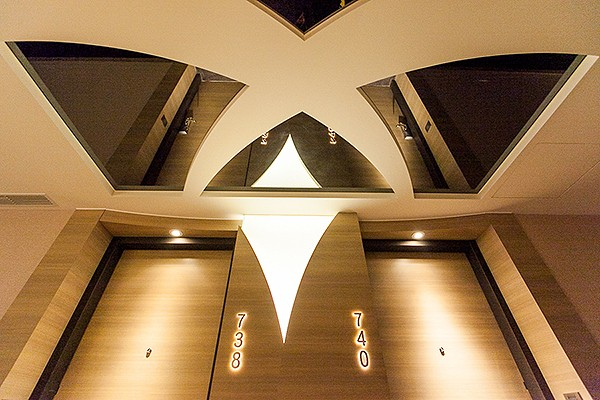環境
•環境
•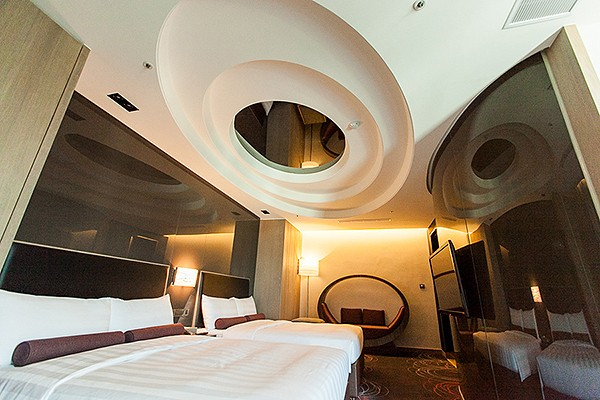環境
•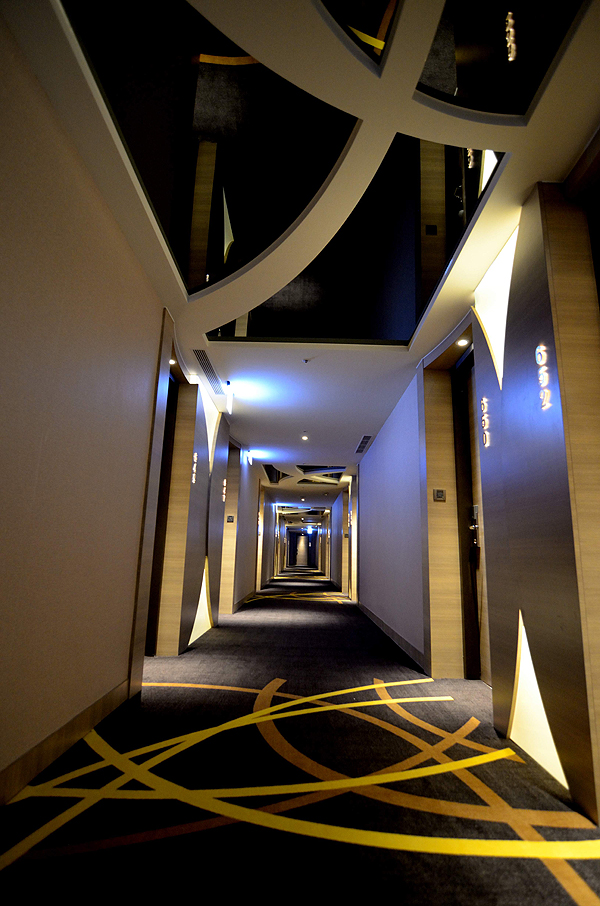環境
•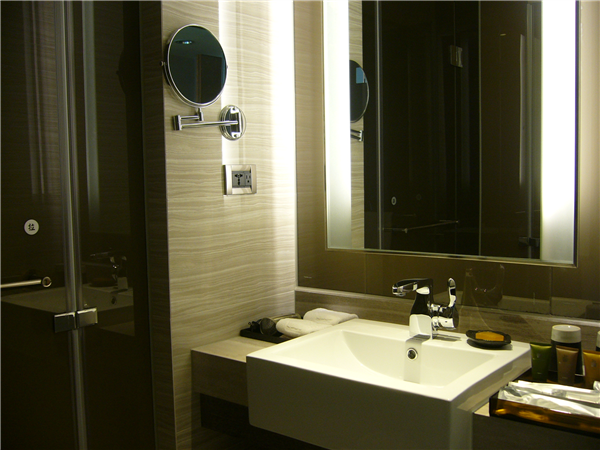客房
•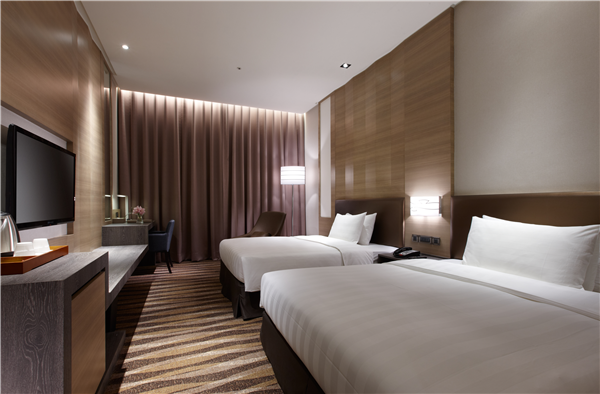客房
•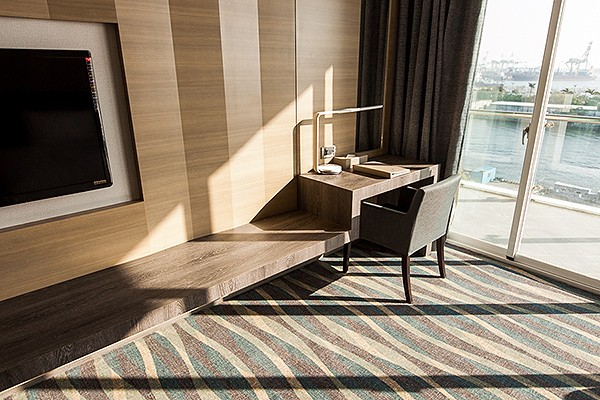環境
•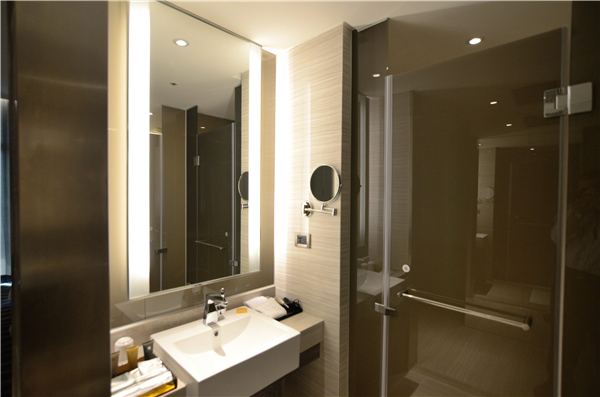客房
•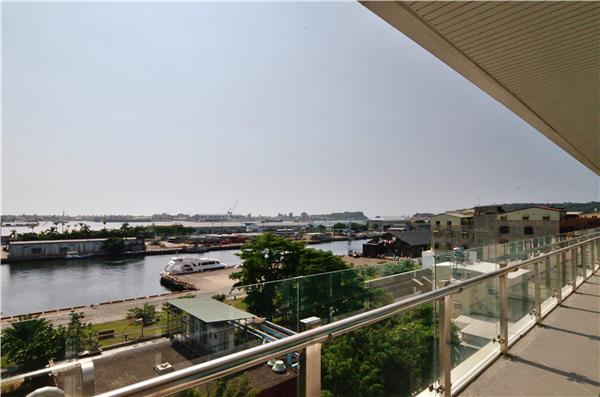客房
•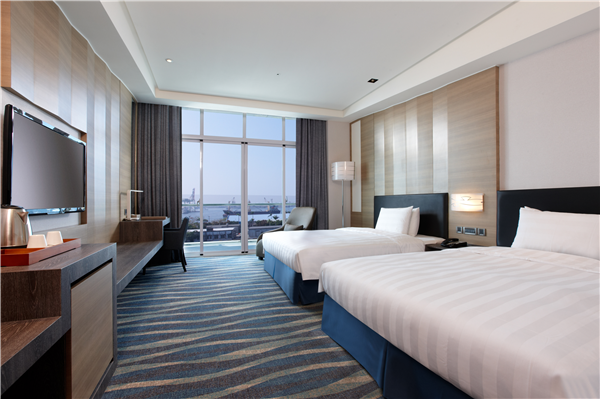客房
•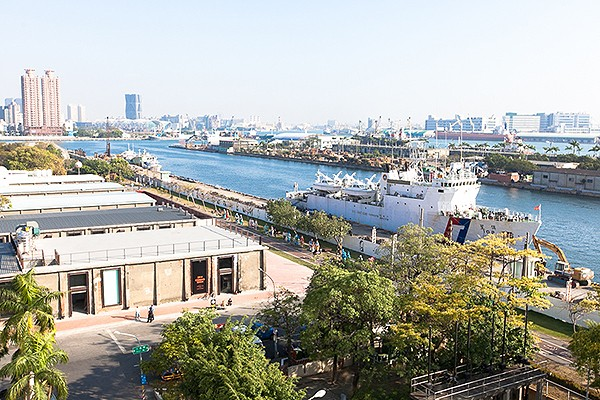環境
•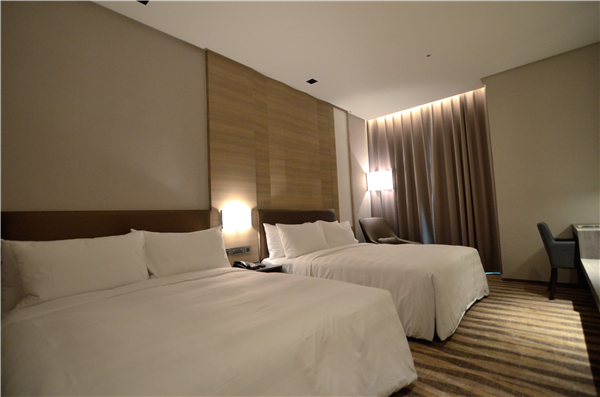客房
•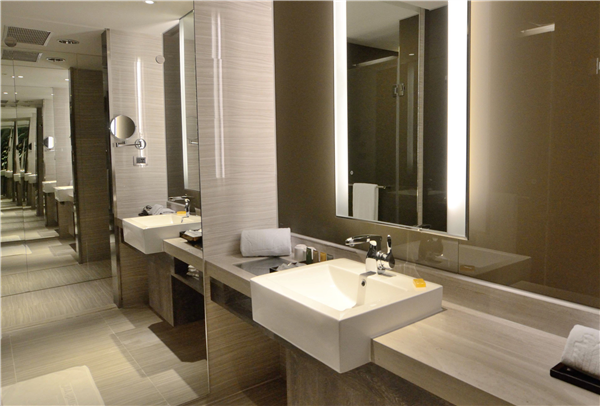客房
•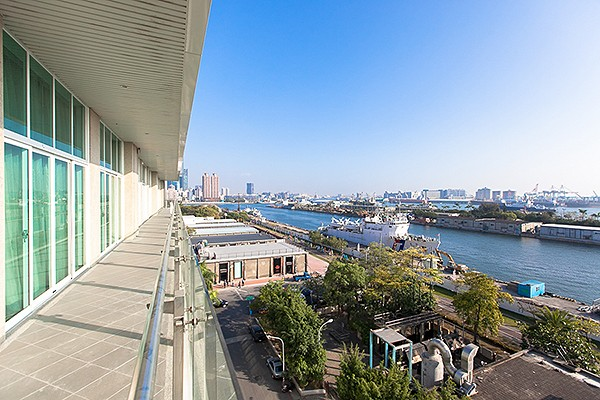環境
•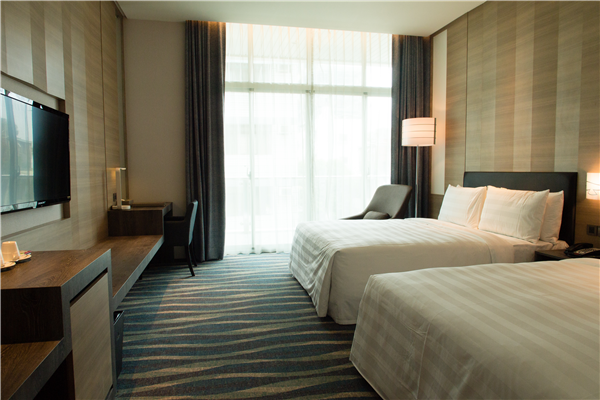客房
•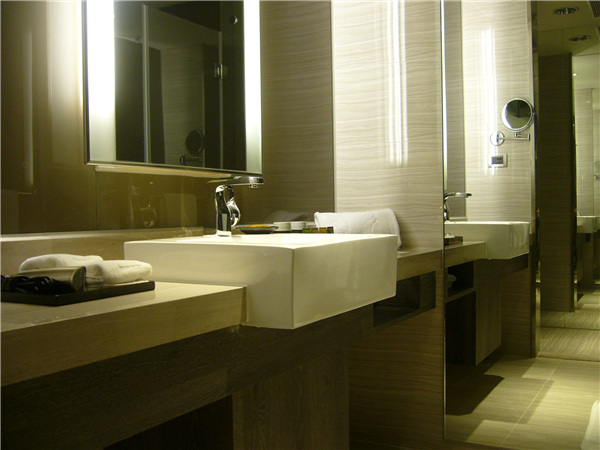客房
•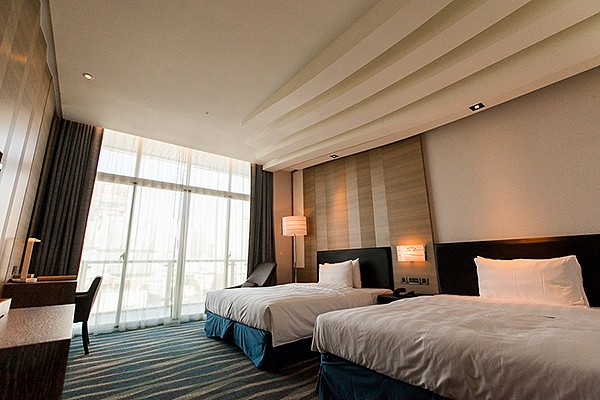環境
•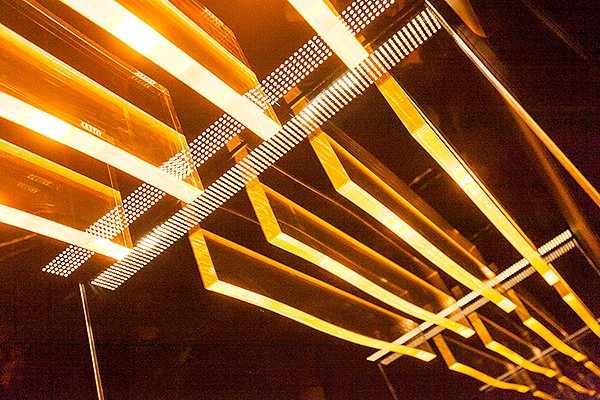環境
•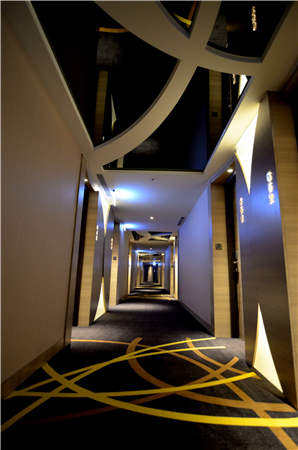酒店內部
•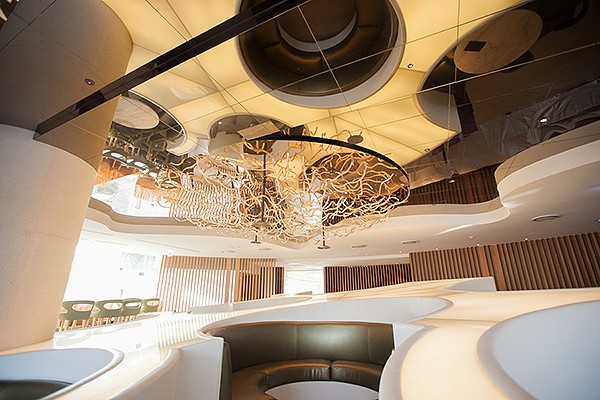環境
•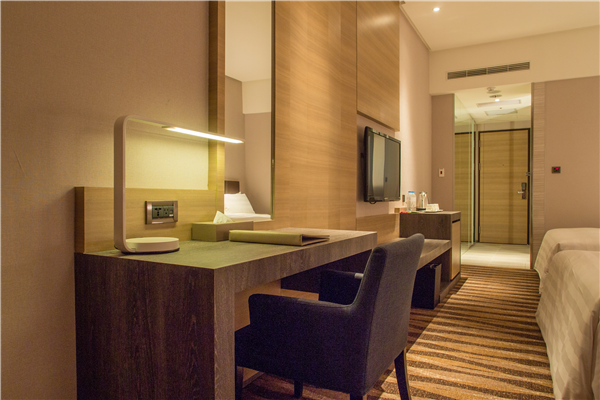客房
•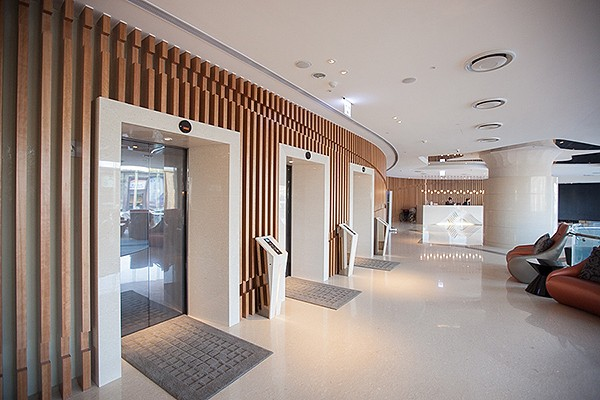環境
•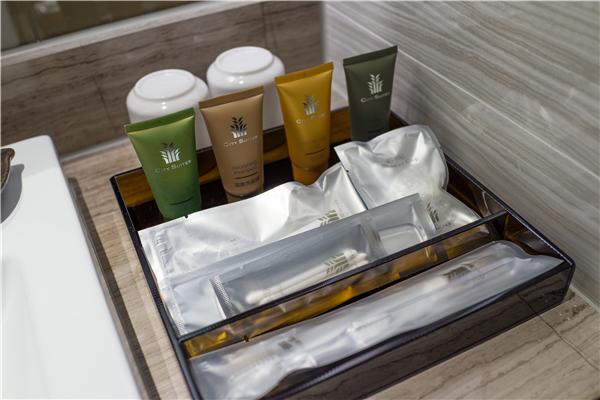酒店內部
•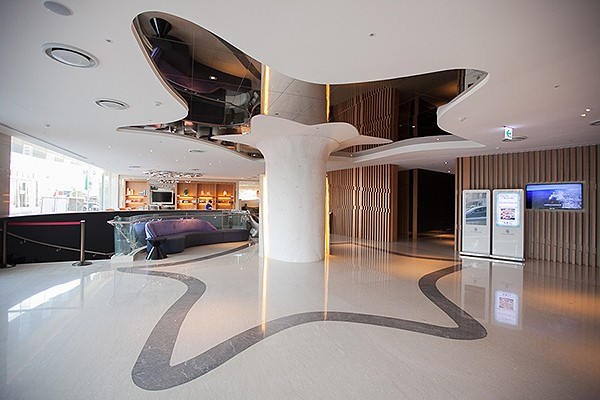環境
•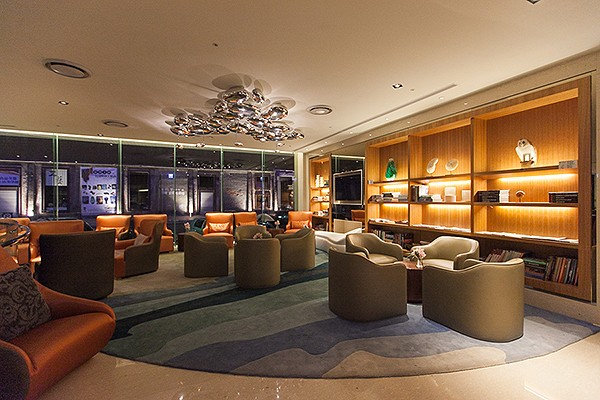環境
•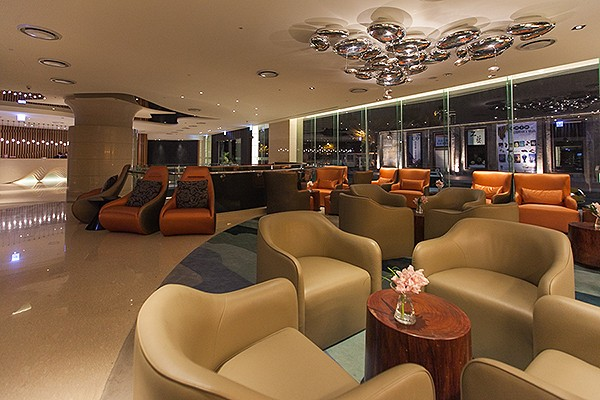環境
•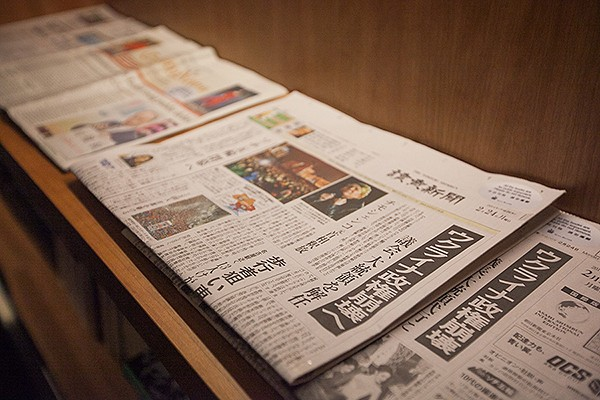環境
•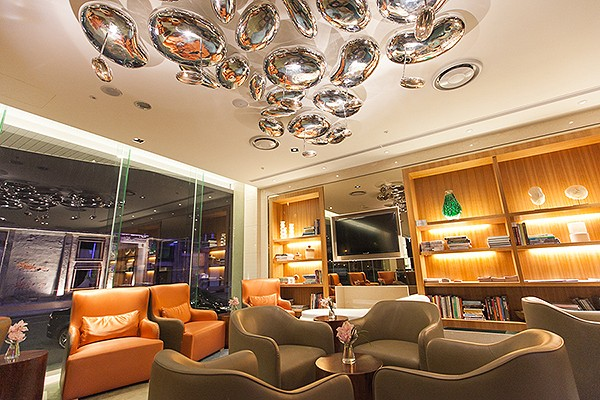環境
•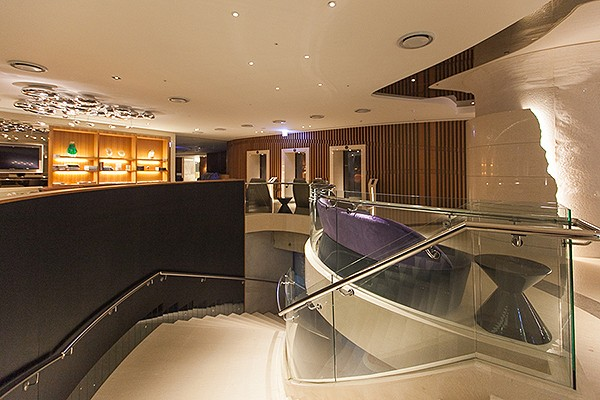環境
•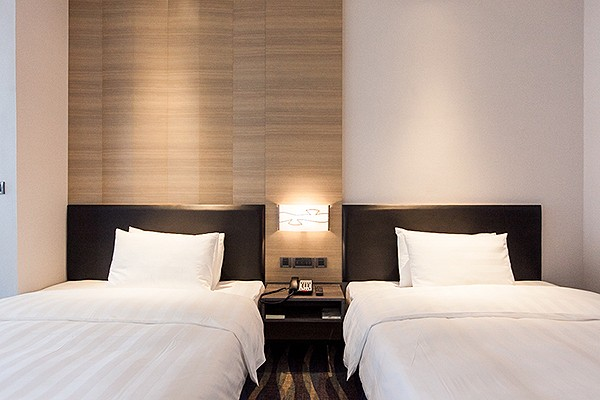環境
•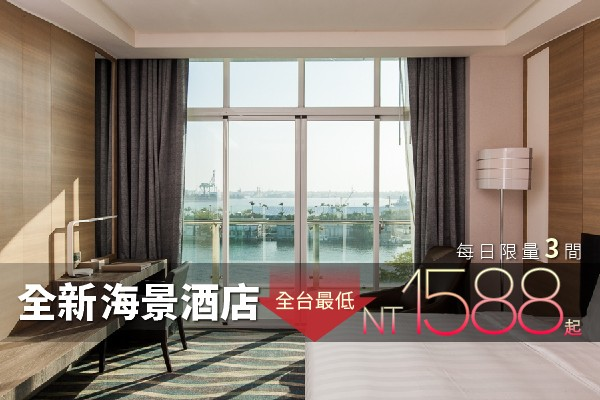環境
•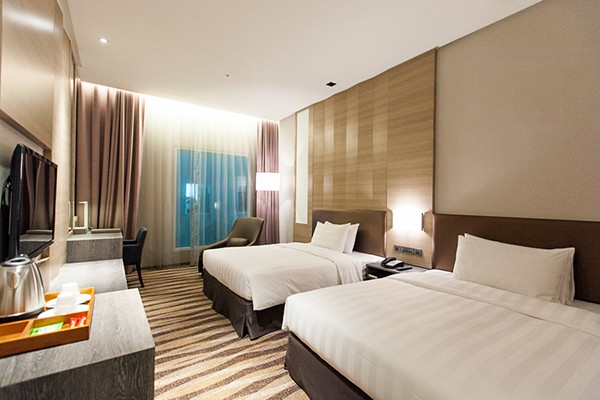客房
•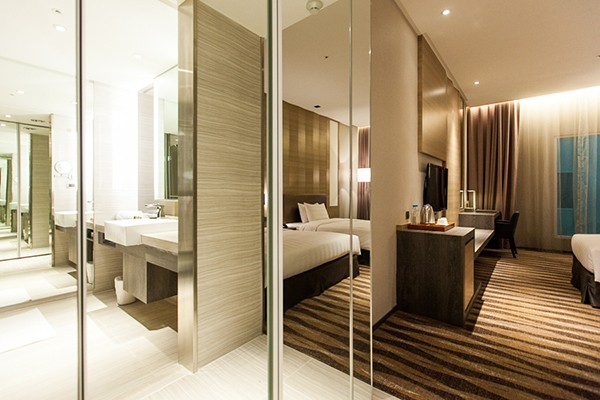客房
•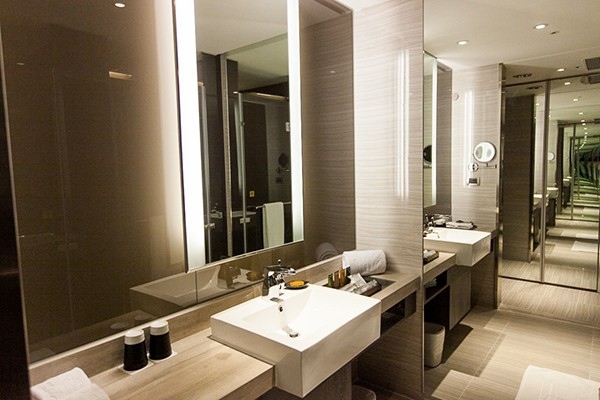客房
•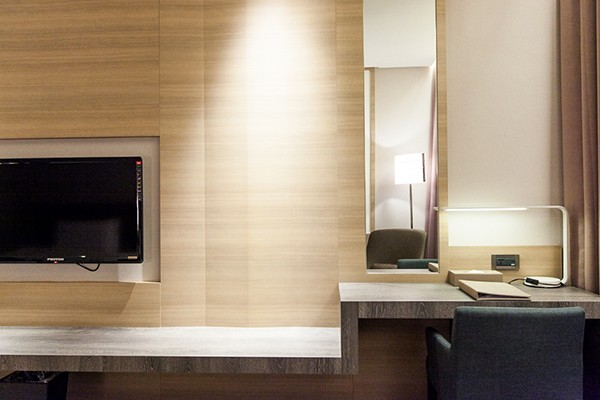客房
•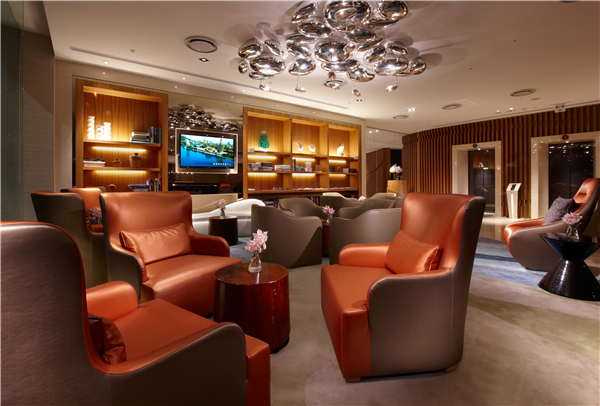商務中心
•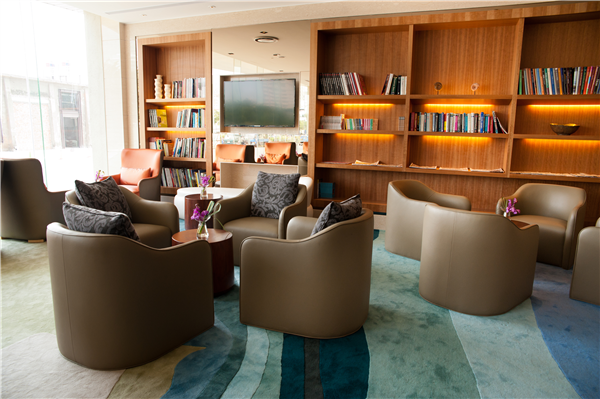商務中心
•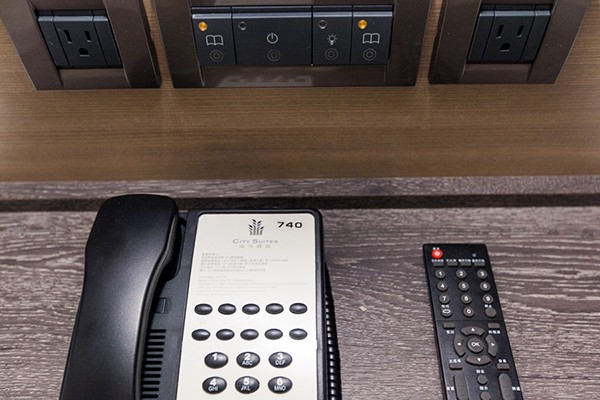客房
•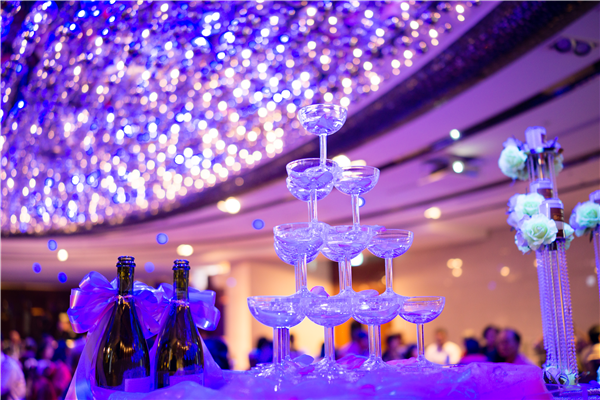宴會廳
•客房
•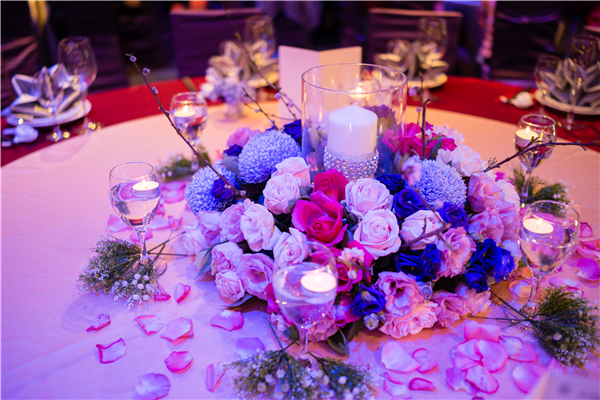宴會廳
•客房
•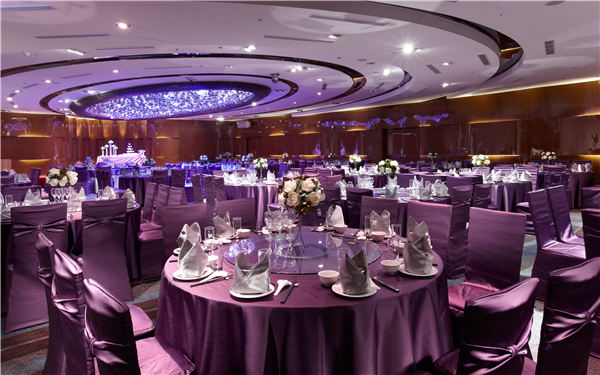宴會廳
•客房
•客房
•客房
•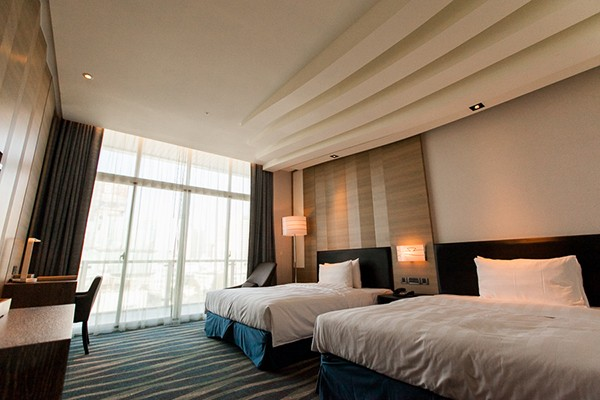客房
•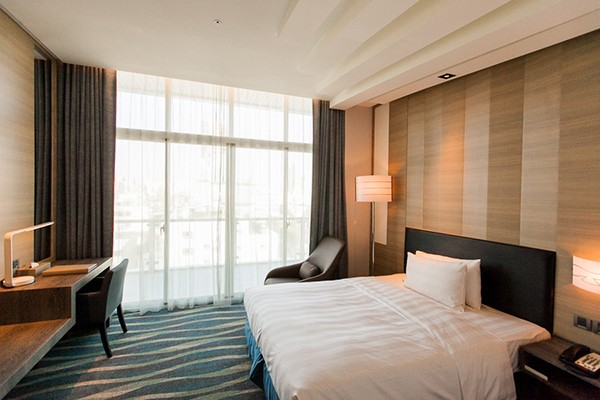客房
•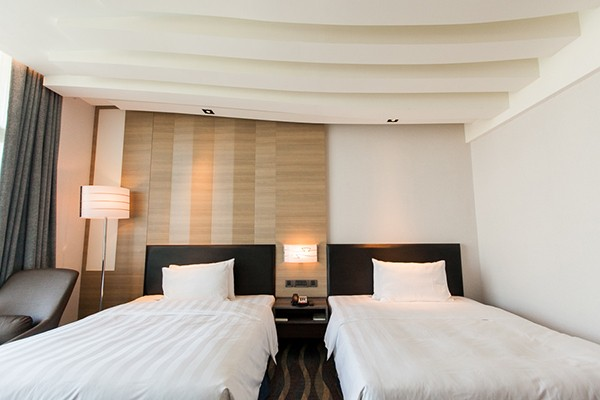客房
•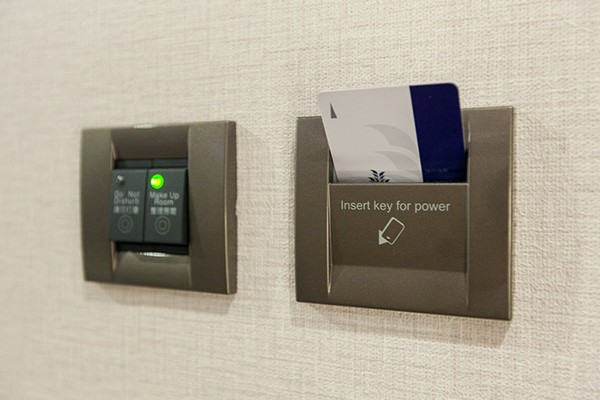客房
•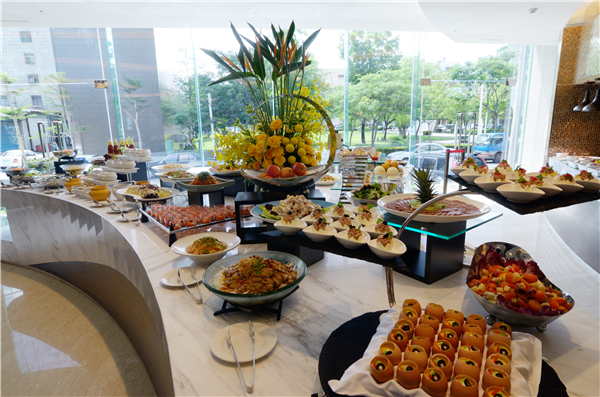自助餐
•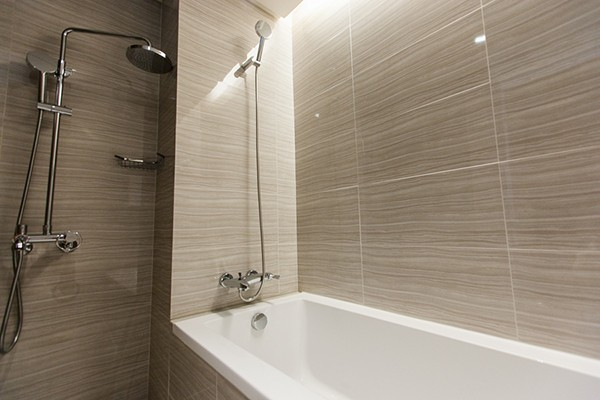客房
•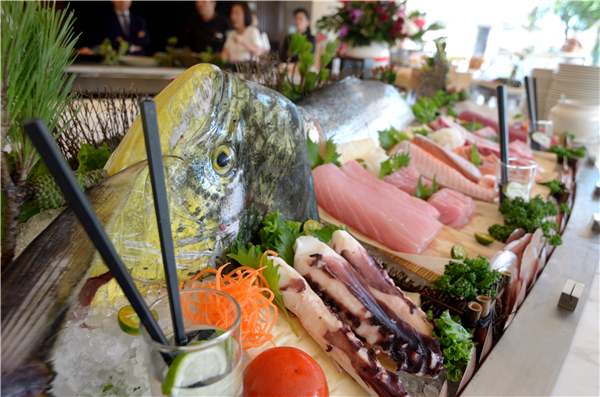自助餐
•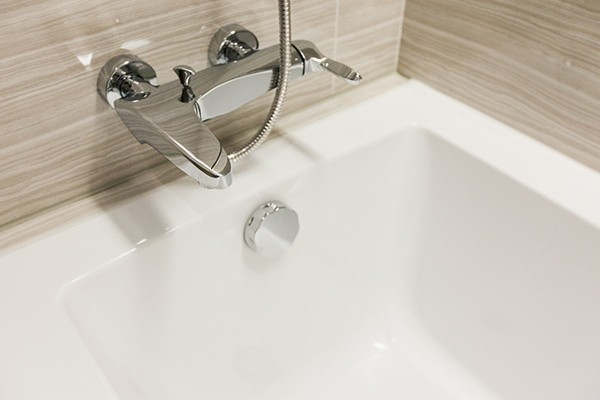客房
•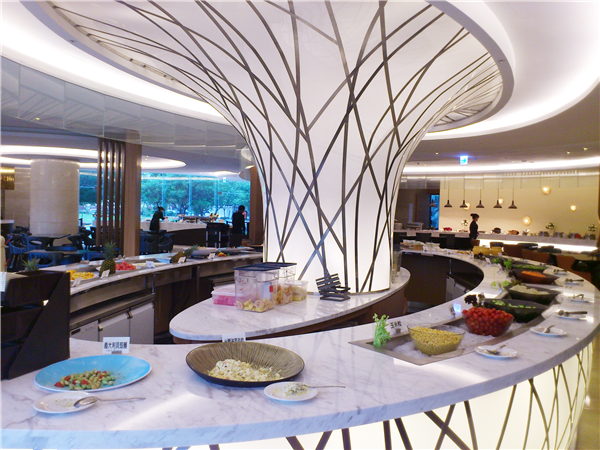自助餐
•客房
•客房
•客房
•客房
•客房
•客房
•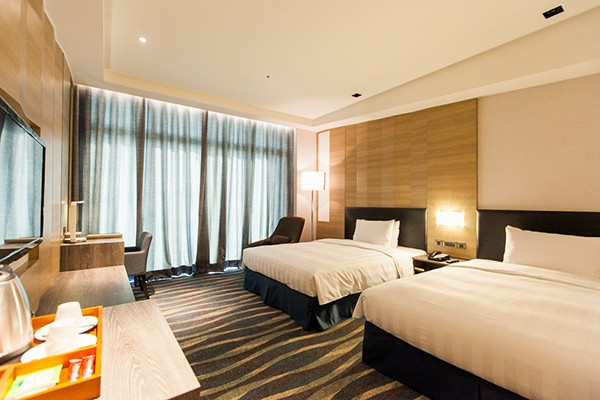客房
•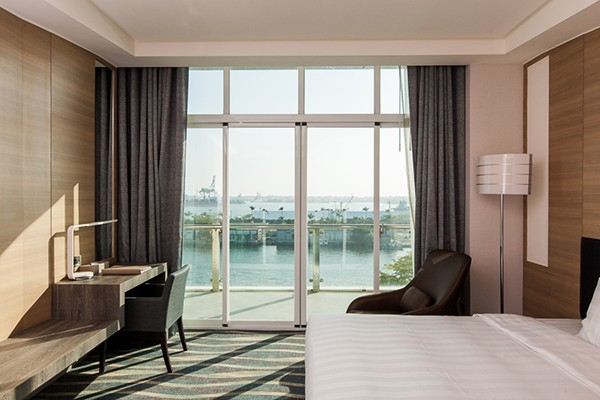客房
•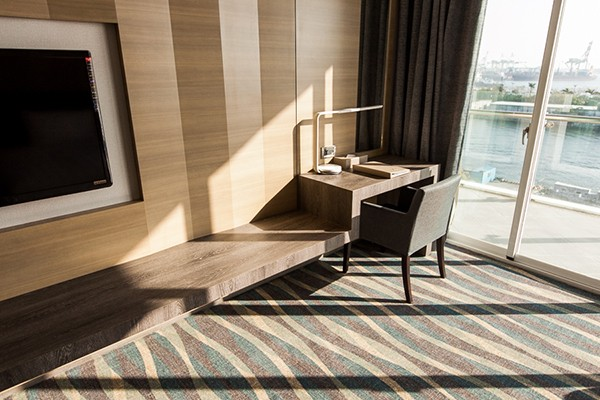客房
•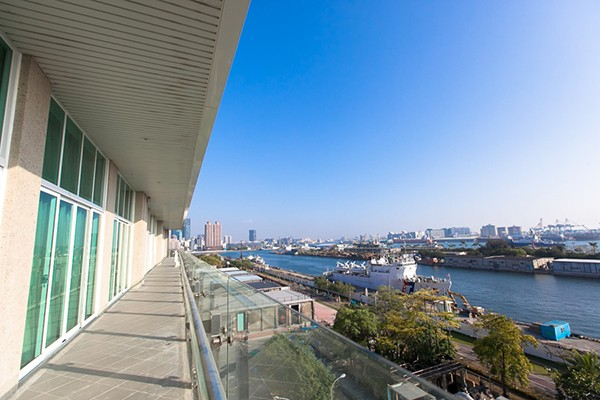客房
•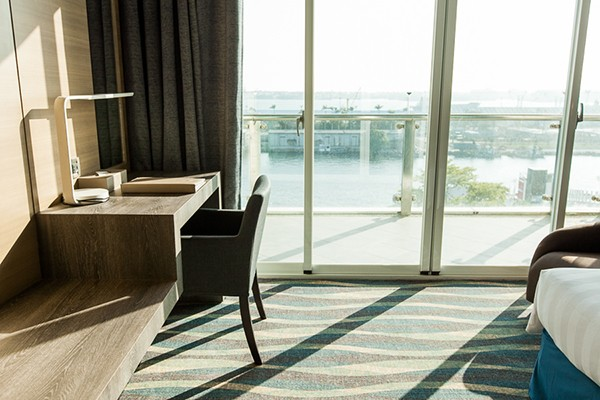客房
•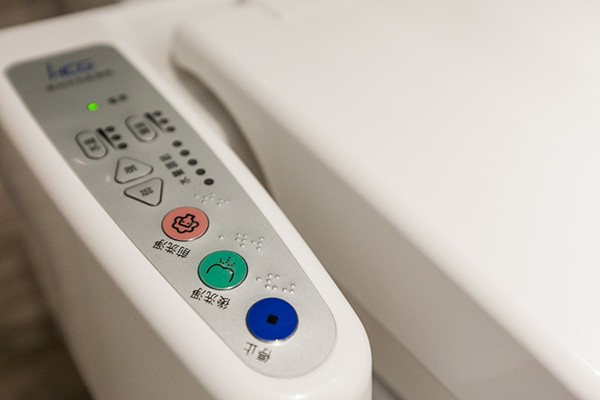客房
•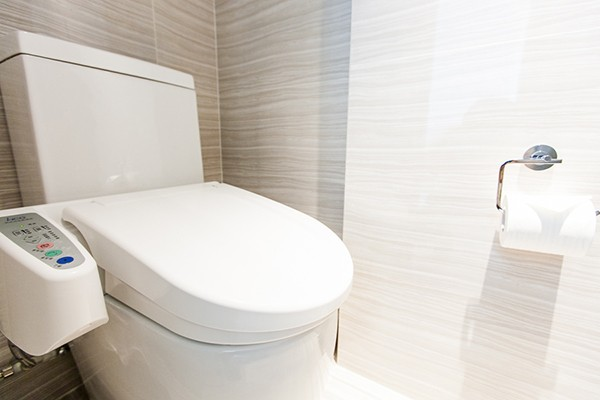客房
•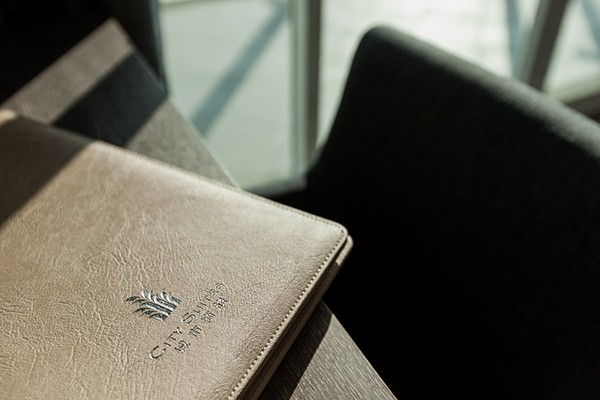客房
•客房
•客房
•客房
•客房
•客房
•客房
•客房
•客房

#### 飯店設有　 飯店未設 飯店設施

• 24小時客房服務
• 商務中心
• 洗衣服務/乾洗
• Wi-Fi in public areas
• 夜總會
• 禮賓接待服務
• 代客泊車
• 娛樂場
• 自行車出租服務
• 保管服務
• 客房服務
• 行政樓層
• 停車場
• 家庭房
• 酒吧
• 兒童看護服務
• 接送服務
• 電梯
• 可帶寵物
• 旅遊服務
• 飯店/機場接送
• 吸煙房
• 會議設施
• 餐廳
• 咖啡店
• 殘障人士設施
• 髮廊
• 商店
• 池畔吧

• DVD/CD播放器
• 小廚房
• 無線上網(收費)
• 休憩區
• 微波爐
• 燙衣板
• 免費瓶裝水
• 房內保險箱
• 獨立淋浴間
• 公共浴室
• 房內遊戲機
• 獨立用餐區
• 冰箱
• 手提電腦保險箱
• 禁煙房
• 冷氣
• 按摩浴缸
• 私人泳池
• 加長的床(>2m)
• 日報
• 茶與咖啡沖泡設備
• 可提供相連房間
• 書桌
• 衛星頻道/有線電視
• 吹風機
• 洗衣機
• 迷你吧
• 寬頻上網
• 浴室
• 陽台/露台
• 寬頻上網(免費)
• 浴缸
• 電影點播服務
• 寬頻上網(收費)
• 浴袍
• 電視
• 寬頻上網(無線)
• 液晶電視
• 電風扇
• 尊用休息室
• 無線上網(免費)

• spa
• 三溫暖/水療設備
• 網球場
• 健身房/健身設施
• 水上活動
• 花園
• 兒童娛樂室
• 水上運動項目(非機動項目)
• 蒸浴室
• 壁球場
• 溫泉
• 遊戲室/娛樂場
• 室內游泳池
• 游泳池(兒童)
• 高爾夫球場(3km內)
• 室外游泳池
• 漩渦按摩浴池
• 高爾夫球場(飯店)
• 按摩
• 私人海灘

#### 飯店簡介

```『高雄真愛館』緊鄰真愛碼頭及駁二藝術特區，近捷運鹽埕埔站(橘線O2)，無論是前往城市光廊、英國領事館、旗津或歷史博物館等地皆相當便利。在真愛館可欣賞大樓林立的現代都市風貌，也可眺望海灣體驗大船入港的震撼，絕佳的視野讓您感受白天與夜晚不同的風貌，享受於港都城市中特別的住房體驗。

```

#### 飯店條款

• 加床政策根據您所選定的客房而有所不同，更多詳情請查看您所選定的客房的政策

#### 相關資訊

電梯 Yes Yes Yes 0Km 298間 Yes 330TWD 9.5Km 7 329TWD 1 12:00 PM 3:00 PM 30分鐘 110V 2014

0
0